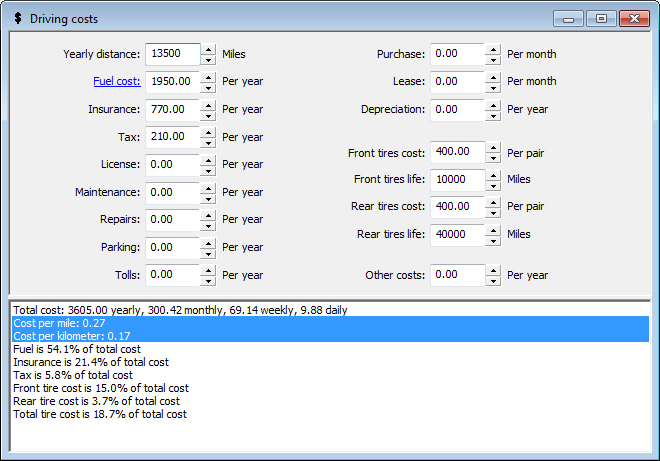## Track Your Driving Costs

• Find out exactly how much you spend to operate your vehicle.
• See how much each individual expense adds to the total.
• Use fuel costs and driving distance from the fuel costs calculation or enter any numbers you want.Enter your driving distance and fuel and other vehicle costs. Calculate your total driving cost, cost per mile, cost per kilometer, fuel percentage of total cost, insurance percentage of total cost, tax percentage of total cost, front tire percentage of total cost, rear tire percentage of total cost, and overall tire percentage of total cost.